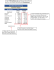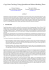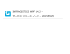# MS Excel Exercise 1: Formulas & Functions

## Transcription

MS Excel Exercise 1: Formulas & Functions
```MS Excel Exercise 1: Formulas & Functions
Create a Spreadsheet that calculates statistics regarding the number of students enrolled at
Armstrong Twp. High School during a 5 year time period.
1. Begin by opening up a new worksheet in Microsoft Excel.
2. Copy the following spreadsheet exactly as show below. Make sure that you copy the exact
statistics shown on the table. Use the same rows and columns as shown.
3. The Highlighted Cells are where you will need to enter your formulas.
4. For each school year, enter a formula that will ADD the total number of males and females
5. Enter a formula that calculates the Average Total Number of students by grade level for each
of the 5 years.
6. Enter formulas that calculate the Total Number of Students enrolled at ATHS for each of the
5 years.
7. Use the following equation to create formulas that calculate the percentage of students in each
grade level, for each of the 5 years.
Total # of Students in a Grade Level / Total # of Students = % of Students in Grade Level
8. Format the Percentages with NO DECIMALS!
9. Create OUTSIDE BORDERS around all of your cells where you have entered formulas
10. Save your work in your Excel folder Chapter 11 as Review_Ex.1_yourname
MS Excel Exercise 2: Space Weight
1. You will be constructing a spreadsheet that will calculate your current weight if you were
standing (and still alive!) on each of the planets (including Pluto).
2. Open a New Worksheet in Microsoft
Excel.
3. Type the spreadsheet shown to the right.
4. In the column titled, New Weight (lbs)
insert a formula that multiplies the gravity
factor by the weight entered in the cell
entitled, My Weight (lbs). MAKE SURE
TO USE ABSOLUTE REFERENCING!!!
5. After you have entered your formulas and
planet. Highlight the entire table of planets,
gravity factors and new weight, and Sort the
Data by Gravity Factor in Ascending order.
7. Test your weight calculator by typing in different weights in the cell next to the My Weight
(lbs) = . You should notice that the New Weight column adjusts automatically. If not, check
8. Type your full name in row 1.
9. Save the workbook in your excel folder Chapter 11 as Review_Ex.2_yourname.
MS Excel Exercise 3: GPA Calculator
1. You will be constructing a spreadsheet that you can use to calculate your current Grade Point
Average. (GPA)
2. Open a New Worksheet in Microsoft Excel.
3. Copy the spreadsheet shown to the next page.
Under the heading Subject, list the classes that you currently are enrolled in.
4. In the column titled, Current Grade,
each subject and enter them in the
appropriate cell.
5. Use an IF Function to create the Grade
Values. Use the NOTE: Grade Values to
Condition: IF Current Grade = “A”, then
4.0, else IF Current Grade = “B”, then 3.0,
else IF Current Grade = “C”, then 2.0, else
IF Current Grade = “D”, then 1.0, else IF
Current Grade = “F”, then 0.0, else 0.0
6. In the Total Values = cell, insert a
formula that calculates the Total of all
individual letter grade values found in the
7. In the MY GPA= cell, insert a formula
that calculates the AVERAGE of all the
individual Grade Values. You can do this
either by using the Function Button and selecting AVERAGE for the range of cells or by
entering a formula for calculating averages. (Total of all values / total # of individual values).
8. Use the Decrease Decimal button to round of the decimals to the nearest 100th. (Two
decimal places)
9. Under the cell that calculates MY GPA, Insert an IF Statement for the following condition:
If a student's GPA is greater than or equal to a 3.50, then have the cell display HONOR
ROLL! If the student's GPA is less than the 3.50, have the cell display, Keep Trying!
10. Try out your GPA Calculator. Type in a series of low grades (1's & 2's) and then high
grades (3's & 4's) and see if the IF statement changes to Keep Trying or Honor Roll!
11. TYPE YOUR FULL NAME IN ROW 1
12. Save the workbook in your excel folder Chapter 11 as Review_Ex.3_yourname
```

### VISUAL FACTORY FOR EXCEL Aaron Evans October 11, 2012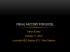### UCSB COLLABORATE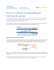### NeueNeue LL öö sungsanssungsans ää tzetze durchdurch Excel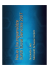### MICROSOFT EXCEL Introduction to Microsoft Excel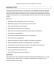### Sample Exam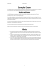### here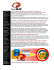### File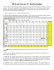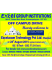### Ms. EL-Shahat - Elizabeth Blackwell Middle School 210Q / Homepage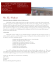### How to Read My Transcript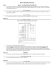### EL1-U1-A4-CMDeptBudgets(A4,Step5)